# 最大流

## Ford-Fulkerson 增广路算法

### 增广路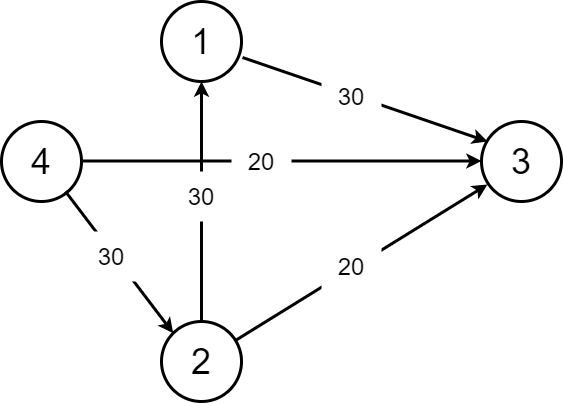1. 这条 增广路 的总流量为 。到 的时候还是 ，到 了就只有 了。

2. 这样子我们就很好的保留了 的流量。

### Edmonds-Karp 动能算法（EK 算法）

1. 找？我们就从源点一直 BFS 走来走去，碰到汇点就停，然后增广（每一条路都要增广）。我们在 BFS 的时候就注意一下流量合不合法就可以了。

2. 增广？其实就是按照我们找的增广路在重新走一遍。走的时候把这条路的能够成的最大流量减一减，然后给答案加上最小流量就可以了。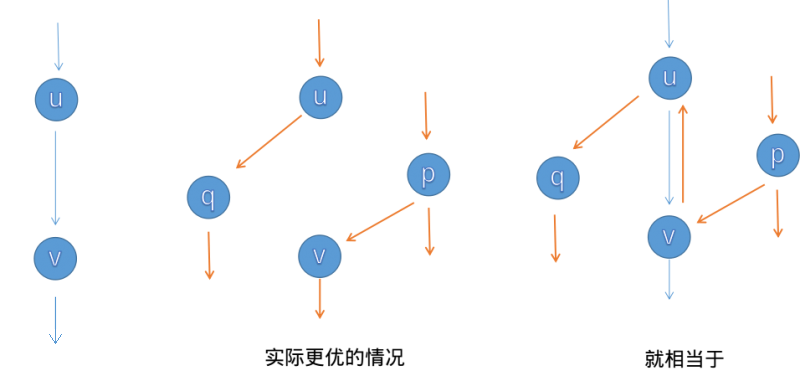EK 算法的时间复杂度为 （其中 为点数， 为边数）。效率还有很大提升空间。

  1 2 3 4 5 6 7 8 9 10 11 12 13 14 15 16 17 18 19 20 21 22 23 24 25 26 27 28 29 30 31 32 33 34 35 36 37 38 39 40 41 42 43 44 45 46 47 48 49 50 51 52 53 54 55 56 57 58 59 60 61 62 #define maxn 250 #define INF 0x3f3f3f3f struct Edge { int from, to, cap, flow; Edge(int u, int v, int c, int f) : from(u), to(v), cap(c), flow(f) {} }; struct EK { int n, m; // n：点数，m：边数 vector edges; // edges：所有边的集合 vector G[maxn]; // G：点 x -> x 的所有边在 edges 中的下标 int a[maxn], p[maxn]; // a：点 x -> BFS 过程中最近接近点 x 的边给它的最大流 // p：点 x -> BFS 过程中最近接近点 x 的边 void init(int n) { for (int i = 0; i < n; i++) G[i].clear(); edges.clear(); } void AddEdge(int from, int to, int cap) { edges.push_back(Edge(from, to, cap, 0)); edges.push_back(Edge(to, from, 0, 0)); m = edges.size(); G[from].push_back(m - 2); G[to].push_back(m - 1); } int Maxflow(int s, int t) { int flow = 0; for (;;) { memset(a, 0, sizeof(a)); queue Q; Q.push(s); a[s] = INF; while (!Q.empty()) { int x = Q.front(); Q.pop(); for (int i = 0; i < G[x].size(); i++) { // 遍历以 x 作为起点的边 Edge& e = edges[G[x][i]]; if (!a[e.to] && e.cap > e.flow) { p[e.to] = G[x][i]; // G[x][i] 是最近接近点 e.to 的边 a[e.to] = min(a[x], e.cap - e.flow); // 最近接近点 e.to 的边赋给它的流 Q.push(e.to); } } if (a[t]) break; // 如果汇点接受到了流，就退出 BFS } if (!a[t]) break; // 如果汇点没有接受到流，说明源点和汇点不在同一个连通分量上 for (int u = t; u != s; u = edges[p[u]].from) { // 通过 u 追寻 BFS 过程中 s -> t 的路径 edges[p[u]].flow += a[t]; // 增加路径上边的 flow 值 edges[p[u] ^ 1].flow -= a[t]; // 减小反向路径的 flow 值 } flow += a[t]; } return flow; } }; 

### Dinic 算法

Dinic 算法 的过程是这样的：每次增广前，我们先用 BFS 来将图分层。设源点的层数为 ，那么一个点的层数便是它离源点的最近距离。

1. 如果不存在到汇点的增广路（即汇点的层数不存在），我们即可停止增广。
2. 确保我们找到的增广路是最短的。（原因见下文）

Dinic 算法有两个优化：

1. 多路增广：每次找到一条增广路的时候，如果残余流量没有用完怎么办呢？我们可以利用残余部分流量，再找出一条增广路。这样就可以在一次 DFS 中找出多条增广路，大大提高了算法的效率。
2. 当前弧优化：如果一条边已经被增广过，那么它就没有可能被增广第二次。那么，我们下一次进行增广的时候，就可以不必再走那些已经被增广过的边。

#### 时间复杂度

  1 2 3 4 5 6 7 8 9 10 11 12 13 14 15 16 17 18 19 20 21 22 23 24 25 26 27 28 29 30 31 32 33 34 35 36 37 38 39 40 41 42 43 44 45 46 47 48 49 50 51 52 53 54 55 56 57 58 59 60 61 62 63 64 65 66 67 68 69 70 71 72 73 74 75 76 77 #define maxn 250 #define INF 0x3f3f3f3f struct Edge { int from, to, cap, flow; Edge(int u, int v, int c, int f) : from(u), to(v), cap(c), flow(f) {} }; struct Dinic { int n, m, s, t; vector edges; vector G[maxn]; int d[maxn], cur[maxn]; bool vis[maxn]; void init(int n) { for (int i = 0; i < n; i++) G[i].clear(); edges.clear(); } void AddEdge(int from, int to, int cap) { edges.push_back(Edge(from, to, cap, 0)); edges.push_back(Edge(to, from, 0, 0)); m = edges.size(); G[from].push_back(m - 2); G[to].push_back(m - 1); } bool BFS() { memset(vis, 0, sizeof(vis)); queue Q; Q.push(s); d[s] = 0; vis[s] = 1; while (!Q.empty()) { int x = Q.front(); Q.pop(); for (int i = 0; i < G[x].size(); i++) { Edge& e = edges[G[x][i]]; if (!vis[e.to] && e.cap > e.flow) { vis[e.to] = 1; d[e.to] = d[x] + 1; Q.push(e.to); } } } return vis[t]; } int DFS(int x, int a) { if (x == t || a == 0) return a; int flow = 0, f; for (int& i = cur[x]; i < G[x].size(); i++) { Edge& e = edges[G[x][i]]; if (d[x] + 1 == d[e.to] && (f = DFS(e.to, min(a, e.cap - e.flow))) > 0) { e.flow += f; edges[G[x][i] ^ 1].flow -= f; flow += f; a -= f; if (a == 0) break; } } return flow; } int Maxflow(int s, int t) { this->s = s; this->t = t; int flow = 0; while (BFS()) { memset(cur, 0, sizeof(cur)); flow += DFS(s, INF); } return flow; } }; 

### MPM 算法

MPM(Malhotra, Pramodh-Kumar and Maheshwari) 算法得到最大流的方式有两种：使用基于堆的优先队列，时间复杂度为 ；常用 BFS 解法，时间复杂度为 。注意，本章节只专注于分析更优也更简洁的 算法。

MPM 算法的整体结构和 Dinic 算法类似，也是分阶段运行的。在每个阶段，在 的残量网络的分层网络中找到增广路。它与 Dinic 算法的主要区别在于寻找增广路的方式不同：MPM 算法中寻找增广路的部分的只花了 , 时间复杂度要优于 Dinic 算法。

MPM 算法需要考虑顶点而不是边的容量。在分层网络 中，如果定义点 的容量 为其传入残量和传出残量的最小值，则有：

#### 时间复杂度分析

MPM 算法的每个阶段都需要 ，因为最多有 次迭代（因为至少删除了所选的参考节点），并且在每次迭代中，我们删除除最多 之外经过的所有边。求和，我们得到 。由于阶段总数少于 ，因此 MPM 算法的总运行时间为

##### 阶段总数小于 V 的证明

MPM 算法在少于 个阶段内结束。为了证明这一点，我们必须首先证明两个引理。

  1 2 3 4 5 6 7 8 9 10 11 12 13 14 15 16 17 18 19 20 21 22 23 24 25 26 27 28 29 30 31 32 33 34 35 36 37 38 39 40 41 42 43 44 45 46 47 48 49 50 51 52 53 54 55 56 57 58 59 60 61 62 63 64 65 66 67 68 69 70 71 72 73 74 75 76 77 78 79 80 81 82 83 84 85 86 87 88 89 90 91 92 93 94 95 96 97 98 99 100 101 102 103 104 105 106 107 108 109 110 111 112 113 114 115 116 117 118 119 120 121 122 123 124 125 126 127 128 129 130 131 132 133 134 135 136 137 138 139 140 141 142 143 144 145 146 147 148 149 150 151 152 153 154 155 156 157 158 159 160 161 162 163 164 165 166 167 168 169 170 171 172 173 174 175 176 177 178 179 struct MPM { struct FlowEdge { int v, u; long long cap, flow; FlowEdge() {} FlowEdge(int _v, int _u, long long _cap, long long _flow) : v(_v), u(_u), cap(_cap), flow(_flow) {} FlowEdge(int _v, int _u, long long _cap) : v(_v), u(_u), cap(_cap), flow(0ll) {} }; const long long flow_inf = 1e18; vector edges; vector alive; vector pin, pout; vector > in, out; vector > adj; vector ex; int n, m = 0; int s, t; vector level; vector q; int qh, qt; void resize(int _n) { n = _n; ex.resize(n); q.resize(n); pin.resize(n); pout.resize(n); adj.resize(n); level.resize(n); in.resize(n); out.resize(n); } MPM() {} MPM(int _n, int _s, int _t) { resize(_n); s = _s; t = _t; } void add_edge(int v, int u, long long cap) { edges.push_back(FlowEdge(v, u, cap)); edges.push_back(FlowEdge(u, v, 0)); adj[v].push_back(m); adj[u].push_back(m + 1); m += 2; } bool bfs() { while (qh < qt) { int v = q[qh++]; for (int id : adj[v]) { if (edges[id].cap - edges[id].flow < 1) continue; if (level[edges[id].u] != -1) continue; level[edges[id].u] = level[v] + 1; q[qt++] = edges[id].u; } } return level[t] != -1; } long long pot(int v) { return min(pin[v], pout[v]); } void remove_node(int v) { for (int i : in[v]) { int u = edges[i].v; auto it = find(out[u].begin(), out[u].end(), i); out[u].erase(it); pout[u] -= edges[i].cap - edges[i].flow; } for (int i : out[v]) { int u = edges[i].u; auto it = find(in[u].begin(), in[u].end(), i); in[u].erase(it); pin[u] -= edges[i].cap - edges[i].flow; } } void push(int from, int to, long long f, bool forw) { qh = qt = 0; ex.assign(n, 0); ex[from] = f; q[qt++] = from; while (qh < qt) { int v = q[qh++]; if (v == to) break; long long must = ex[v]; auto it = forw ? out[v].begin() : in[v].begin(); while (true) { int u = forw ? edges[*it].u : edges[*it].v; long long pushed = min(must, edges[*it].cap - edges[*it].flow); if (pushed == 0) break; if (forw) { pout[v] -= pushed; pin[u] -= pushed; } else { pin[v] -= pushed; pout[u] -= pushed; } if (ex[u] == 0) q[qt++] = u; ex[u] += pushed; edges[*it].flow += pushed; edges[(*it) ^ 1].flow -= pushed; must -= pushed; if (edges[*it].cap - edges[*it].flow == 0) { auto jt = it; ++jt; if (forw) { in[u].erase(find(in[u].begin(), in[u].end(), *it)); out[v].erase(it); } else { out[u].erase(find(out[u].begin(), out[u].end(), *it)); in[v].erase(it); } it = jt; } else break; if (!must) break; } } } long long flow() { long long ans = 0; while (true) { pin.assign(n, 0); pout.assign(n, 0); level.assign(n, -1); alive.assign(n, true); level[s] = 0; qh = 0; qt = 1; q = s; if (!bfs()) break; for (int i = 0; i < n; i++) { out[i].clear(); in[i].clear(); } for (int i = 0; i < m; i++) { if (edges[i].cap - edges[i].flow == 0) continue; int v = edges[i].v, u = edges[i].u; if (level[v] + 1 == level[u] && (level[u] < level[t] || u == t)) { in[u].push_back(i); out[v].push_back(i); pin[u] += edges[i].cap - edges[i].flow; pout[v] += edges[i].cap - edges[i].flow; } } pin[s] = pout[t] = flow_inf; while (true) { int v = -1; for (int i = 0; i < n; i++) { if (!alive[i]) continue; if (v == -1 || pot(i) < pot(v)) v = i; } if (v == -1) break; if (pot(v) == 0) { alive[v] = false; remove_node(v); continue; } long long f = pot(v); ans += f; push(v, s, f, false); push(v, t, f, true); alive[v] = false; remove_node(v); } } return ans; } }; 

### ISAP

  1 2 3 4 5 6 7 8 9 10 11 12 13 14 15 16 17 18 19 20 21 22 23 24 25 26 27 28 29 30 31 32 33 34 35 36 37 38 39 40 41 42 43 44 45 46 47 48 49 50 51 52 53 54 55 56 57 58 59 60 61 62 63 64 65 66 67 68 69 70 71 72 73 74 75 76 77 78 79 80 81 82 83 84 85 86 87 88 89 90 91 92 93 94 95 96 97 98 99 100 101 102 103 104 105 106 107 108 109 110 111 struct Edge { int from, to, cap, flow; Edge(int u, int v, int c, int f) : from(u), to(v), cap(c), flow(f) {} }; bool operator<(const Edge& a, const Edge& b) { return a.from < b.from || (a.from == b.from && a.to < b.to); } struct ISAP { int n, m, s, t; vector edges; vector G[maxn]; bool vis[maxn]; int d[maxn]; int cur[maxn]; int p[maxn]; int num[maxn]; void AddEdge(int from, int to, int cap) { edges.push_back(Edge(from, to, cap, 0)); edges.push_back(Edge(to, from, 0, 0)); m = edges.size(); G[from].push_back(m - 2); G[to].push_back(m - 1); } bool BFS() { memset(vis, 0, sizeof(vis)); queue Q; Q.push(t); vis[t] = 1; d[t] = 0; while (!Q.empty()) { int x = Q.front(); Q.pop(); for (int i = 0; i < G[x].size(); i++) { Edge& e = edges[G[x][i] ^ 1]; if (!vis[e.from] && e.cap > e.flow) { vis[e.from] = 1; d[e.from] = d[x] + 1; Q.push(e.from); } } } return vis[s]; } void init(int n) { this->n = n; for (int i = 0; i < n; i++) G[i].clear(); edges.clear(); } int Augment() { int x = t, a = INF; while (x != s) { Edge& e = edges[p[x]]; a = min(a, e.cap - e.flow); x = edges[p[x]].from; } x = t; while (x != s) { edges[p[x]].flow += a; edges[p[x] ^ 1].flow -= a; x = edges[p[x]].from; } return a; } int Maxflow(int s, int t) { this->s = s; this->t = t; int flow = 0; BFS(); memset(num, 0, sizeof(num)); for (int i = 0; i < n; i++) num[d[i]]++; int x = s; memset(cur, 0, sizeof(cur)); while (d[s] < n) { if (x == t) { flow += Augment(); x = s; } int ok = 0; for (int i = cur[x]; i < G[x].size(); i++) { Edge& e = edges[G[x][i]]; if (e.cap > e.flow && d[x] == d[e.to] + 1) { ok = 1; p[e.to] = G[x][i]; cur[x] = i; x = e.to; break; } } if (!ok) { int m = n - 1; for (int i = 0; i < G[x].size(); i++) { Edge& e = edges[G[x][i]]; if (e.cap > e.flow) m = min(m, d[e.to]); } if (--num[d[x]] == 0) break; num[d[x] = m + 1]++; cur[x] = 0; if (x != s) x = edges[p[x]].from; } } return flow; } }; 

## Push-Relabel 预流推进算法

### 通用的预流推进算法

，称结点 溢出4，注意当我们提到溢出结点时，并不包括

#### 通用算法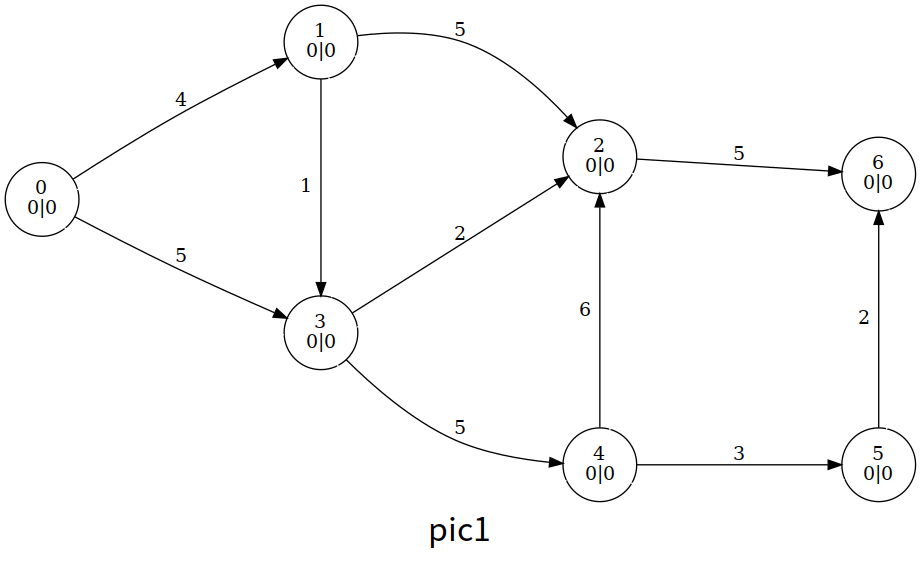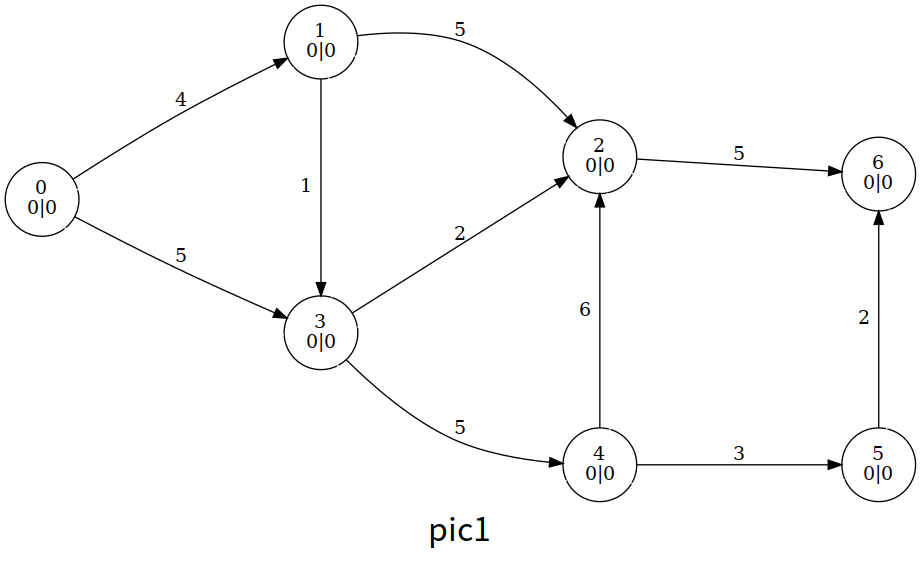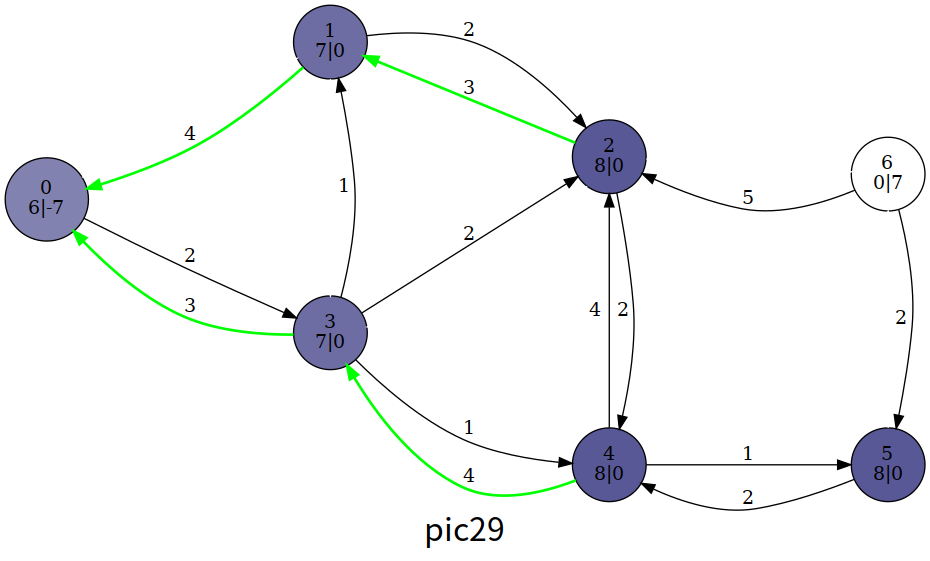1 2 3 4 5 6 7 8 9 10 11 12 13 14 15 16 17 18 19 20 21 22 23 24 25 const int N = 1e4 + 4, M = 1e5 + 5, INF = 0x3f3f3f3f; int n, m, s, t, maxflow, tot; int ht[N], ex[N]; void init() { // 初始化 for (int i = h[s]; i; i = e[i].nex) { const int &v = e[i].t; ex[v] = e[i].v, ex[s] -= ex[v], e[i ^ 1].v = e[i].v, e[i].v = 0; } ht[s] = n; } bool push(int ed) { const int &u = e[ed ^ 1].t, &v = e[ed].t; int flow = min(ex[u], e[ed].v); ex[u] -= flow, ex[v] += flow, e[ed].v -= flow, e[ed ^ 1].v += flow; return ex[u]; // 如果 u 仍溢出，返回 1 } void relabel(int u) { ht[u] = INF; for (int i = h[u]; i; i = e[i].nex) if (e[i].v) ht[u] = min(ht[u], ht[e[i].t]); ++ht[u]; } 

### HLPP 算法

1. 初始化（基于预流推进算法）；
2. 选择溢出结点中高度最高的结点 ，并对它所有可以推送的边进行推送；
3. 如果 仍溢出，对它重贴标签，回到步骤 2；
4. 如果没有溢出的结点，算法结束。

#### BFS 优化

HLPP 的上界为 ，但在使用时卡得比较紧；我们可以在初始化高度的时候进行优化。具体来说，我们初始化 的最短距离；特别地，

#### GAP 优化

HLPP 推送的条件是 ，而如果在算法的某一时刻， 的结点个数为 ，那么对于 的结点就永远无法推送超额流到 ，因此只能送回 ，那么我们就在这时直接让他们的高度变成至少 ，以尽快推送回 ，减少重贴标签的操作。

LuoguP4722【模板】最大流 加强版/预流推进
  1 2 3 4 5 6 7 8 9 10 11 12 13 14 15 16 17 18 19 20 21 22 23 24 25 26 27 28 29 30 31 32 33 34 35 36 37 38 39 40 41 42 43 44 45 46 47 48 49 50 51 52 53 54 55 56 57 58 59 60 61 62 63 64 65 66 67 68 69 70 71 72 73 74 75 76 77 78 79 80 81 82 83 84 85 86 87 88 89 90 91 92 93 94 95 96 97 98 99 100 101 102 103 104 #include #include #include #include using namespace std; const int N = 1200, M = 120000, INF = 0x3f3f3f3f; int n, m, s, t; struct qxx { int nex, t, v; }; qxx e[M * 2 + 1]; int h[N + 1], cnt = 1; void add_path(int f, int t, int v) { e[++cnt] = (qxx){h[f], t, v}, h[f] = cnt; } void add_flow(int f, int t, int v) { add_path(f, t, v); add_path(t, f, 0); } int ht[N + 1], ex[N + 1], gap[N]; // 高度; 超额流; gap 优化 gap[i] 为高度为 i 的节点的数量 stack B[N]; // 桶 B[i] 中记录所有 ht[v]==i 的v int level = 0; // 溢出节点的最高高度 int push(int u) { // 尽可能通过能够推送的边推送超额流 bool init = u == s; // 是否在初始化 for (int i = h[u]; i; i = e[i].nex) { const int &v = e[i].t, &w = e[i].v; if (!w || init == false && ht[u] != ht[v] + 1) // 初始化时不考虑高度差为1 continue; int k = init ? w : min(w, ex[u]); // 取到剩余容量和超额流的最小值，初始化时可以使源的溢出量为负数。 if (v != s && v != t && !ex[v]) B[ht[v]].push(v), level = max(level, ht[v]); ex[u] -= k, ex[v] += k, e[i].v -= k, e[i ^ 1].v += k; // push if (!ex[u]) return 0; // 如果已经推送完就返回 } return 1; } void relabel(int u) { // 重贴标签（高度） ht[u] = INF; for (int i = h[u]; i; i = e[i].nex) if (e[i].v) ht[u] = min(ht[u], ht[e[i].t]); if (++ht[u] < n) { // 只处理高度小于 n 的节点 B[ht[u]].push(u); level = max(level, ht[u]); ++gap[ht[u]]; // 新的高度，更新 gap } } bool bfs_init() { memset(ht, 0x3f, sizeof(ht)); queue q; q.push(t), ht[t] = 0; while (q.size()) { // 反向 BFS, 遇到没有访问过的结点就入队 int u = q.front(); q.pop(); for (int i = h[u]; i; i = e[i].nex) { const int &v = e[i].t; if (e[i ^ 1].v && ht[v] > ht[u] + 1) ht[v] = ht[u] + 1, q.push(v); } } return ht[s] != INF; // 如果图不连通，返回 0 } // 选出当前高度最大的节点之一, 如果已经没有溢出节点返回 0 int select() { while (B[level].size() == 0 && level > -1) level--; return level == -1 ? 0 : B[level].top(); } int hlpp() { // 返回最大流 if (!bfs_init()) return 0; // 图不连通 memset(gap, 0, sizeof(gap)); for (int i = 1; i <= n; i++) if (ht[i] != INF) gap[ht[i]]++; // 初始化 gap ht[s] = n; push(s); // 初始化预流 int u; while ((u = select())) { B[level].pop(); if (push(u)) { // 仍然溢出 if (!--gap[ht[u]]) for (int i = 1; i <= n; i++) if (i != s && i != t && ht[i] > ht[u] && ht[i] < n + 1) ht[i] = n + 1; // 这里重贴成 n+1 的节点都不是溢出节点 relabel(u); } } return ex[t]; } int main() { scanf("%d%d%d%d", &n, &m, &s, &t); for (int i = 1, u, v, w; i <= m; i++) { scanf("%d%d%d", &u, &v, &w); add_flow(u, v, w); } printf("%d", hlpp()); return 0; }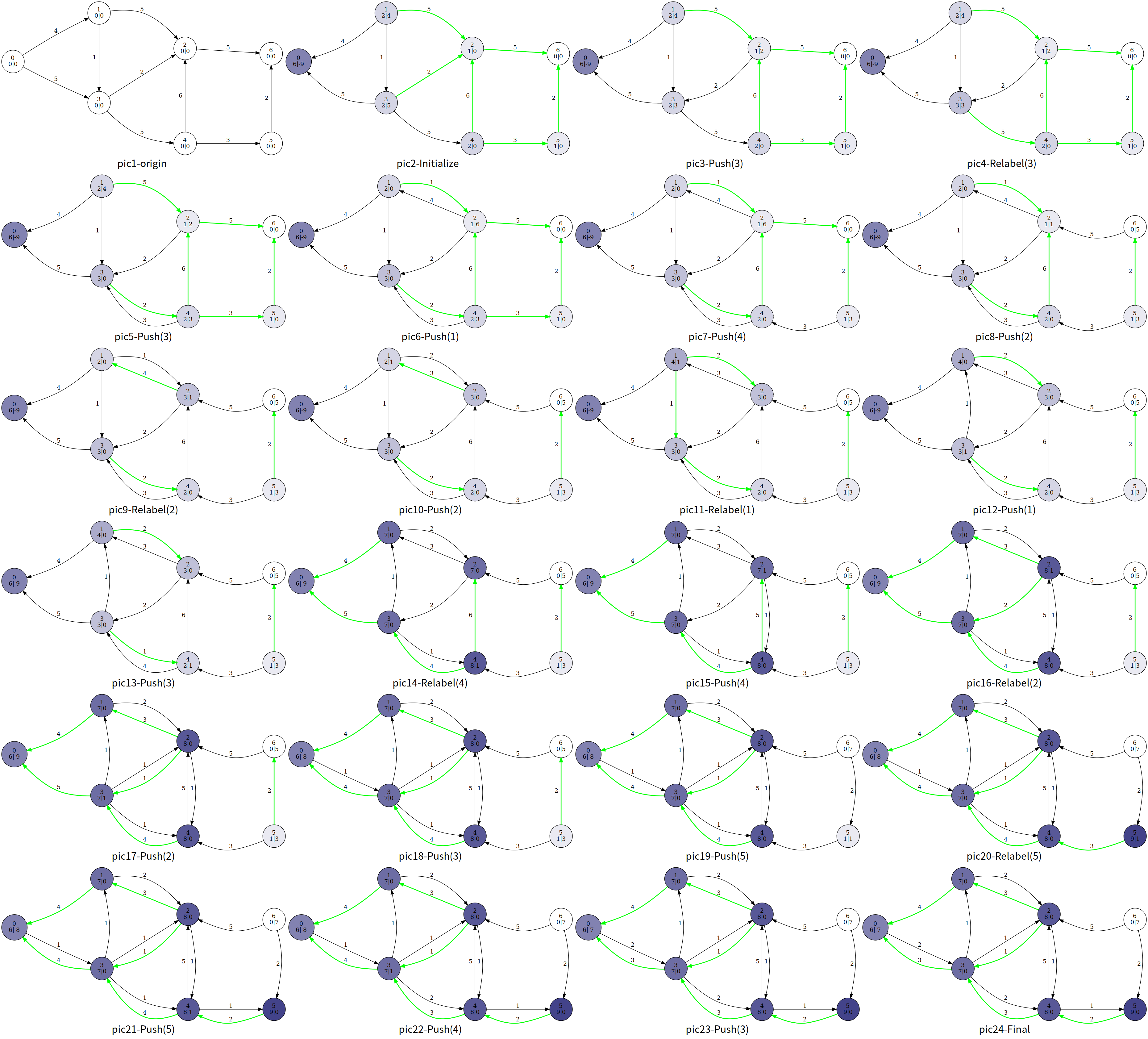## 脚注

1. Cherkassky B V, Goldberg A V. On implementing push-relabel method for the maximum flow problem[C]//International Conference on Integer Programming and Combinatorial Optimization. Springer, Berlin, Heidelberg, 1995: 157-171.

2. Ahuja R K, Kodialam M, Mishra A K, et al. Computational investigations of maximum flow algorithms[J]. European Journal of Operational Research, 1997, 97(3): 509-542.

3. Derigs U, Meier W. Implementing Goldberg's max-flow-algorithm—A computational investigation[J]. Zeitschrift für Operations Research, 1989, 33(6): 383-403.

4. 英语文献中通常称为“active“。

5. 在英语文献中，一个结点的高度通常被称为“distance label”。此处使用的“高度”这个术语源自算法导论中的相关章节。你可以在机械工业出版社算法导论（原书第 3 版）的 P432 脚注中找到这么做的理由。